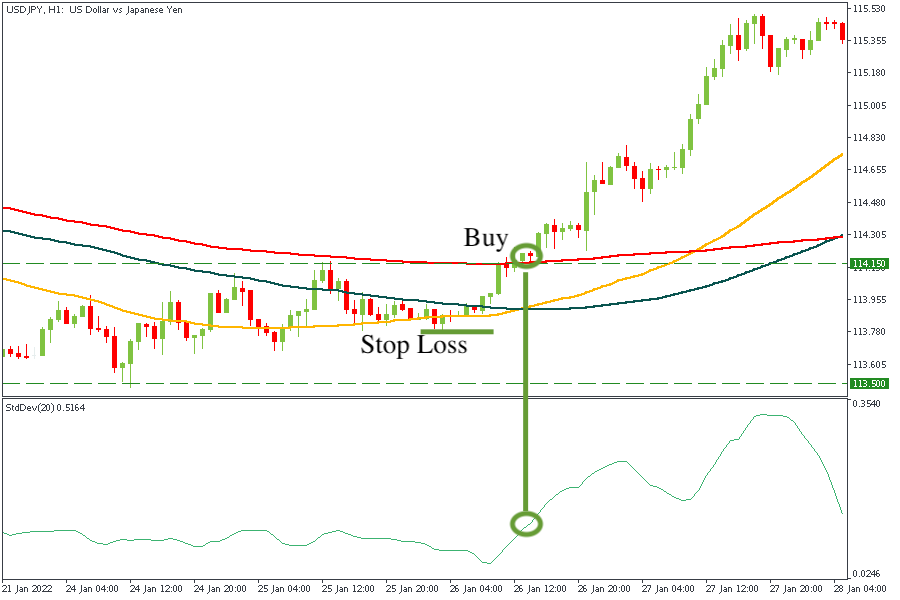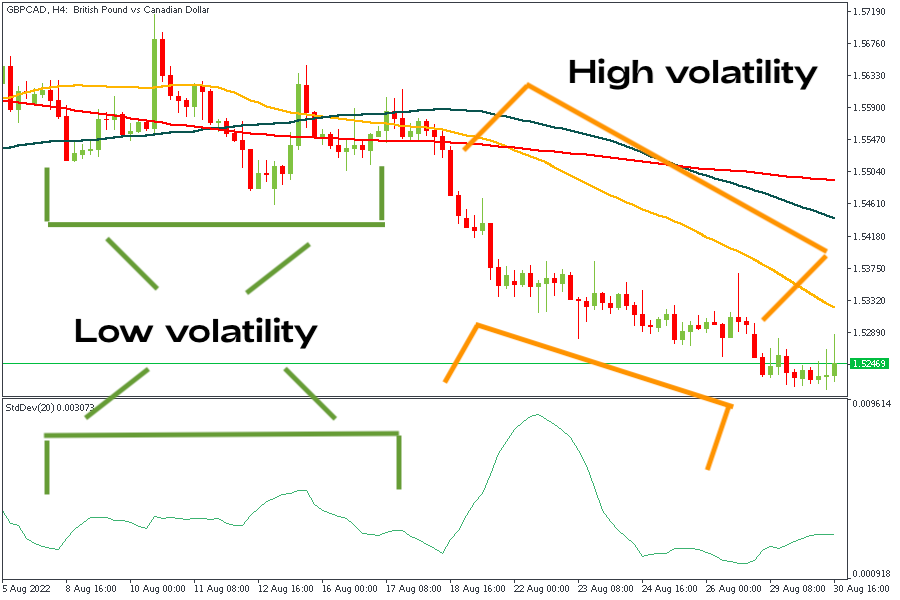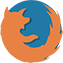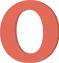The Standard Deviation indicator shows whether volatility is high or low. In this article, we’ll delve into the indicator and explain how it can help you enter the market.

## What is Standard Deviation in trading?

The Standard Deviation indicator is a statistical measure of market volatility. It measures price deviations from the Moving Average. If prices are trading in a narrow trading range, the Standard Deviation indicator returns a low value, indicating low volatility. Conversely, if prices fluctuate sharply up and down, the Standard Deviation returns a high value, indicating high volatility.

## Standard Deviation indicator in MT5

The Standard Deviation indicator is one of the default indicators in MetaTrader. To find the indicator, go to “Insert”, choose “Indicators” and then “Trend”.

## How to calculate Standard Deviation

To calculate Standard Deviation, you need to:

1. Calculate the average of all data points. The result is calculated by adding all data points and dividing them by the number of data points.
2. Calculate the variance for each data point. The variance for each data point is calculated by subtracting the mean from the value of the data point.
3. Square the variance of each data point (from step 2).
4. Sum of squared variance values (from step 3).
5. Divide the sum of squares of the variance values (from step 4) by the number of data points in the data set minus 1.
6. Take the square root of the quotient (from step 5).

Fortunately, MetaTrader does all these calculations for you. You only need to understand the principle of the indicator and interpret its values.

## What is the use of Standard Deviation?

Standard Deviation can be used for three purposes.

First, it’s used to identify outliers. Outliers are anomalies or deviations in a set of data, representing the situations in which good trading opportunities can arise. Identifying outliers can be especially helpful for scalpers and traders who use advanced trading strategies.

Second, the indicator is used to choose the right entry moment based on trends. Standard Deviation helps to understand whether the current prices have deviated enough from the average and, therefore, can return to their average value.

Third, Standard Deviation is used to set an entry point based on outliers when prices point to a narrow trading range, and suddenly strong Standard Deviation pushes prices away from the mean.

For example, you can face the “escape from the range” signal. This signal appears after the quotes leave the range – the correction area before the start of a new movement. Before the price exceeds the range, the indicator line should be at its lows, and you can draw a horizontal level through its nearest highs in the window. When the price goes out of the range, the Standard Deviation line also breaks its level up, confirming the entry signal. On the hourly chart of the USDJPY pair below, we can see:

• The price is rising up from a flat position.
• The line of the indicator confirms the signal rising above the level drawn through its nearest highs, and you can open a buy position.
• Stop Loss is placed under the nearest minimum. A profit can be taken when the price reaches an important resistance level or after signs of a reversal appear.## Standard Deviation vs. variance

Technically, Standard Deviation is the square root of the variance. In turn, the variance is the sum of the squares of the values obtained by subtracting the mean (x) from each value in the data set divided by the number of values in the specified set. Standard Deviation measures the dispersion of returns around the average return.

In other words, Standard Deviation is a measure of volatility. This is a key concept regarding financial risk, viewed as the likelihood that returns will be dispersed around an expected value.

## Pros and cons of Standard Deviation

The Standard Deviation indicator has its own pros and cons. Let’s look at them more closely.

### Pros of Standard Deviation

The Standard Deviation indicator includes all observations. Other deviation measurements, such as range, only measure the most scattered points without considering intermediate points. Therefore, Standard Deviation is often considered a more reliable and accurate measurement than other observations.

Besides, the Standard Deviation of two data sets can be combined using a special combined Standard Deviation formula. There are no similar formulas for other measurements of dispersion observation in statistics. In addition, Standard Deviation can be used in further algebraic calculations, unlike other means of observation.

### Cons of Standard Deviation

Standard Deviation doesn’t measure how far a data point is from the mean. Instead, it compares the square of the difference, the subtle but noticeable difference in the actual variance with the mean.

Besides, outliers have a stronger effect on Standard Deviation. This is especially true when you consider that the difference with the mean is squared, resulting in an even higher number compared to other data points. So remember that Standard Deviation naturally gives more weight to the extreme values.

## How to interpret Standard Deviation

The Standard Deviation shows the scale of price changes relating to the Moving Average. If the indicator value increases, the market is volatile, and the price swings are rather dispersed relative to the moving average. Vice versa, if the indicator value is small, market volatility is low, and the price stays close to the moving average.

Traders need to know that periods of market activity and calm usually alternate each other, and the price tends to return to the average level every time:

• The rise of the Standard Deviation line means high volatility because the closing price and the average closing price significantly differ. Extreme Standard Deviation highs warn that the current activity will soon calm down and will be followed by a period of consolidation.
• The decline of the Standard Deviation line means low volatility and indicates that the market is inactive as prices are stable. Extreme Standard Deviation lows may signal the upcoming market movement.In addition, the current value of Standard Deviation can be used to estimate the significance of a price movement. A movement greater than one Standard Deviation would show above-average strength or weakness of the market, depending on the direction of the movement.

Finally, the Standard Deviation indicator is often used as a part of other more sophisticated indicators, for example, the Bollinger Bands. These bands are set two standard deviations above and below a moving average. Read more about the Bollinger Bands.

## Conclusion

• Pick important market tops or bottoms.
• Target entries within trends.
• Trade with the break if prices are trading in a narrow range and the suddenly high Standard Deviation pushes prices away from the mean.

The Standard Deviation indicator is extremely easy to understand. It shows whether volatility is high or low and helps you enter the market at the right time.

2023-02-16 • Updated

• ## How to open an FBS account?

Click the ‘Open account’ button on our website and proceed to the Personal Area. Before you can start trading, pass a profile verification. Confirm your email and phone number, get your ID verified. This procedure guarantees the safety of your funds and identity. Once you are done with all the checks, go to the preferred trading platform, and start trading.

• ## How to start trading?

If you are 18+ years old, you can join FBS and begin your FX journey. To trade, you need a brokerage account and sufficient knowledge on how assets behave in the financial markets. Start with studying the basics with our free educational materials and creating an FBS account. You may want to test the environment with virtual money with a Demo account. Once you are ready, enter the real market and trade to succeed.

• ## How to withdraw the money you earned with FBS?

The procedure is very straightforward. Go to the Withdrawal page on the website or the Finances section of the FBS Personal Area and access Withdrawal. You can get the earned money via the same payment system that you used for depositing. In case you funded the account via various methods, withdraw your profit via the same methods in the ratio according to the deposited sums.

## Latest news

The Yen Sets New Lows

The Japanese yen fell to its lowest level against the US dollar in 33 years. Read the full report to learn the next target for USDJPY!

Experts are Waiting for US Stocks to Rise

The US stock market fell in the third quarter. What's going on and why economists think that the last quarter will be better? Let's discuss it all here.

BlackRock CEO Supports The USD

BlackRock CEO forecasts the Fed may have to raise rates further. The US dollar index (DXY) gains 130 points today. Read the full report to get more fresh news and technical analysis!

## Data collection notice

FBS maintains a record of your data to run this website. By pressing the “Accept” button, you agree to our Privacy policy.

### Callback

A manager will call you shortly.

A manager will call you shortly.

Next callback request for this phone number
will be available in